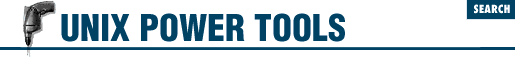home | O'Reilly's CD bookshelfs | FreeBSD | Linux | Cisco | Cisco Exam

#Chapter 46Shell Script Debugging and Gotchas## 46.7 Quoting and Command-Line Parameters

Q: I need to pass a shell script some arguments with multiple words. I thought that putting quotes ( 8.14 ) around command-line arguments would group them. The shell script seems to ignore the quoting, somehow. Here's a simple example:

Q:

```\$ ```

cat script
```

...
for arg in \$*
do
echo "Argument is \$arg"
done
\$ ```

script '1 2 3' 4
```

...
Argument is 1
Argument is 2
Argument is 3
Argument is 4```

A: This is the way ``` \$*``` is defined to work. ``` \$*``` expands to:

A:

`\$1 \$2`

A: [not ``` <">\$1<"> <">\$2<">``` -JP  ] if there are two arguments. Hence the for loop reads:

A:

`for arg in 1 2 3 4`

A: Note that the quotes are gone. What you wanted the shell to see was:

A:

`for arg in '1 2 3' 4`

A: You cannot get that, but you can get something that is Good Enough:

A:

 ``` "\$@" ``` ``` for arg in "\$@"```

A: In effect, ``` \$@``` expands to:

A:

`\$1" "\$2`

A: Putting ``` ""``` s around ``` \$@``` , the effect is:

A:

`for arg in "\$1" "\$2"`

A: Shell quoting is unnecessarily complex. The C shell actually has the right idea (variables can be set to "word lists" ( 47.5 ) ; argv is such a list), but its defaults and syntax for suppressing them make for an artless programming language:

A:

```

foreach arg (\$argv:q)      # colon q ?!?```

A: For the special case of iterating a shell variable over the argument list as it stands at the beginning of the iteration, the Bourne shell provides the construct ``` for arg do``` [i.e., no ``` in ``` ``` list ``` -JP  ]:

A:

```for arg
do echo "Argument is \$arg"
done```

A: produces:

A:

```Argument is 1 2 3
Argument is 4```

A: ``` "\$@"``` is still needed for passing argument lists to other programs. Unfortunately, since ``` \$@``` is defined as expanding to:

A:

```\$1" "\$2...\$```

n-1
```
" "\$```

n
```
```

A: (where ``` n ``` is the number of arguments), when there are no arguments:

A:

`"\$@"`

A: expands to:

A:

`""`

A: and ``` ""``` produces a single argument. [Many UNIX vendors considered this a bug and changed it so that it produces no arguments. -JP  ] The best solution for this is to use, for example:

A:

```% ```

cat bin/okeeffe
```

#! /bin/sh
exec rsh okeeffe.berkeley.edu -l torek \${1+"\$@"}
%```

A: The construct ``` \${1+"\$@"}``` means "expand ``` \$1``` , but if ``` \$1``` is defined, use ``` "\$@"``` instead." [You don't need this on Bourne shells with the "bug fix" I mentioned. -JP  ] Hence, if there are no arguments, we get ``` \$1``` (which is nothing and produces no arguments), otherwise we get ``` "\$@"``` (which expands as above). ``` \${``` ``` var ``` ``` +``` ``` instead ``` ``` }``` is one of several sh \*(lqexpansion shortcuts\*(rq ( 45.12 ) . Another more generally useful one is ``` \${``` ``` var-default ``` ``` }``` , which expands to ``` \$``` ``` var ``` , but if var is not set, to ``` default ``` instead. All of these can be found in the manual for sh , which is worth reading several times, experimenting as you go.

- CT in comp.unix.questions on Usenet, 18 March 198846.6 Watch Out for Bourne Shell -e Bug46.8 Test Built-In Commands for Failure